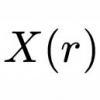# Resources tagged with: Differentiation of parametric and implicit functions

There are 3 NRICH Mathematical resources connected to Differentiation of parametric and implicit functions, you may find related items under Calculus.

Broad Topics > Calculus > Differentiation of parametric and implicit functions### Squareness

##### Age 16 to 18Challenge Level

The family of graphs of x^n + y^n =1 (for even n) includes the circle. Why do the graphs look more and more square as n increases?### Folium of Descartes

##### Age 16 to 18Challenge Level

Investigate the family of graphs given by the equation x^3+y^3=3axy for different values of the constant a.### Implicitly

##### Age 16 to 18Challenge Level

Can you find the maximum value of the curve defined by this expression?